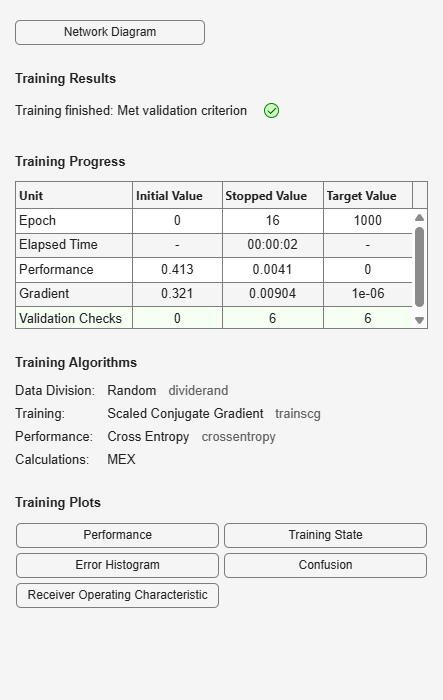# patternnet

Generate pattern recognition network

## Syntax

``net = patternnet(hiddenSizes,trainFcn,performFcn)``

## Description

example

````net = patternnet(hiddenSizes,trainFcn,performFcn)` returns a pattern recognition neural network with a hidden layer size of `hiddenSizes`, a training function, specified by `trainFcn`, and a performance function, specified by `performFcn`.Pattern recognition networks are feedforward networks that can be trained to classify inputs according to target classes. The target data for pattern recognition networks should consist of vectors of all zero values except for a 1 in element `i`, where `i` is the class they are to represent.```

## Examples

collapse all

This example shows how to design a pattern recognition network to classify iris flowers.

`[x,t] = iris_dataset;`

Construct a pattern network with one hidden layer of size 10.

`net = patternnet(10);`

Train the network `net` using the training data.

`net = train(net,x,t);`View the trained network.

`view(net)`Estimate the targets using the trained network.

`y = net(x);`

Assess the performance of the trained network. The default performance function is mean squared error.

`perf = perform(net,t,y)`
```perf = 0.0302 ```
`classes = vec2ind(y);`

## Input Arguments

collapse all

Size of the hidden layers in the network, specified as a row vector. The length of the vector determines the number of hidden layers in the network.

Example: For example, you can specify a network with 3 hidden layers, where the first hidden layer size is 10, the second is 8, and the third is 5 as follows: `[10,8,5]`

The input and output sizes are set to zero. The software adjusts the sizes of these during training according to the training data.

Data Types: `single` | `double`

Training function name, specified as one of the following.

Training FunctionAlgorithm
`'trainlm'`

Levenberg-Marquardt

`'trainbr'`

Bayesian Regularization

`'trainbfg'`

BFGS Quasi-Newton

`'trainrp'`

Resilient Backpropagation

`'trainscg'`

`'traincgb'`

`'traincgf'`

`'traincgp'`

`'trainoss'`

One Step Secant

`'traingdx'`

`'traingdm'`

`'traingd'`

Example: For example, you can specify the variable learning rate gradient descent algorithm as the training algorithm as follows: `'traingdx'`

For more information on the training functions, see Train and Apply Multilayer Shallow Neural Networks and Choose a Multilayer Neural Network Training Function.

Data Types: `char`

Performance function. The default value is `'crossentropy'`.

This argument defines the function used to measure the network’s performance. The performance function is used to calculate network performance during training.

For a list of functions, in the MATLAB command window, type ```help nnperformance```.

## Output Arguments

collapse all

Pattern recognition neural network, returned as a `network` object.

## Version History

Introduced in R2010b